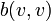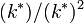# Spinor norm

## Definition

Suppose$k$ is a field,$V$ is a vector space over$k$, and$b$ is a nondegenerate symmetric bilinear form. Let$G$ be the orthogonal group corresponding to$b$. The spinor norm is a homomorphism from$G$ to the multiplicative group modulo squares of$k$:$\! G \to k^*/(k^*)^2$

i.e., it is a homomorphism that sends any element in the orthogonal group to an element in the multiplicative group of$k$ modulo the squares in that group.

The homomorphism is defined as follows: any element of$G$ arising as reflection orthogonal to a vector$v$ is sent to the value$b(v,v)$ modulo$(k^*)^2$. This extends to a well defined and unique homomorphism on all of$G$. For characteristic not equal to 2, the reflection orthogonal to$v$ is defined as:$\! x \mapsto x - 2v\frac{b(v,x)}{b(v,v)}$

For characteristic equal to 2, the reflection orthogonal to$v$ is defined as:$\! x \mapsto x - v\frac{b(v,x)}{b(v,v)}$

Also note that for such a reflection map to exist,$b(v,v)$ must be nonzero, so the map does indeed go to$(k^*)/(k^*)^2$.

Note that different choices of$v$ that are scalar multiples of each other define the same reflection map. That is why the spinor norm is defined only as a map to$k^*/(k^*)^2$ and not as a map to$k^*$.